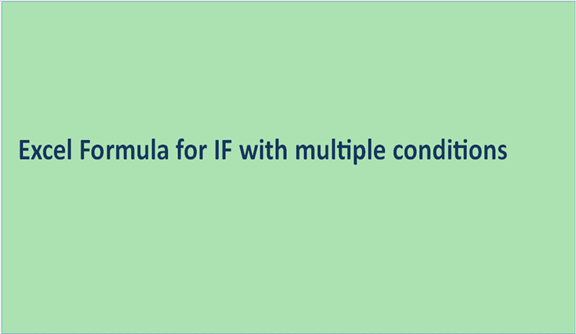## Excel Formula for IF with multiple conditions

The IF function is one of the most commonly used in Excel. The function can be used to test for a single condition as well as check multiple ones for complex logic. We can thus control the execution of Excel tasks using the IF function. This is important since it enables us to perform actions depending on whether they meet certain conditions defined by the IF formula. We can also add data to cells using the function if other cells meet any of the conditions defined in the IF formula. To add multiple conditions to an IF formula, we simply add nested IF functions.

The general syntax for IF function with multiple conditions is

=IF (condition one is true, do something, IF (condition two is true, do something, IF (conditions three is true, do something, else do something)))

Commas are used to separate conditions and IF functions in the IF formula. The ")" is used to close all IFs at the End of the formula while the " (" opens all IF functions. The number of "(" should be equal to that of ")".

1. Basic IF formula that tests a single condition

=IF(D7<=200,"CHEAP")

The if formula above records or posts the String Good whenever the single condition D7<=200.IF formula that checks Two conditions

=IF(D7<=200,"CHEAP","EXPENSIVE")The formula will add the string/text Good when B3>50 else it will post the String/text Bad. It will thus test two conditions.

2. IF formula with multiple conditions.

We can also include multiple conditions in the IF formula as follows

=IF(D7<=200,"CHEAP",IF(D7<=300,"AFFORDABLE",IF(D7<=400,"EXPENSIVE",IF(D7<=500,"VERY EXPENSIVE","LUXURIOUS"))))

For newer versions of Excel, I.e. 2007-present versions, one can nest as many as 64 if functions that can test certain conditions.

To add an IF formula with multiple conditions in Excel:

1. Open Excel. Launch your workbook.

2. Add a column for IF results. This is optional especially if you intend to apply the formula for just a single cell.

3. Enter the If formula in one of the cells.4. Press Enter. Drag the bottom right corner of the cell to automatically apply the formula on other cells.

5. Save changes.